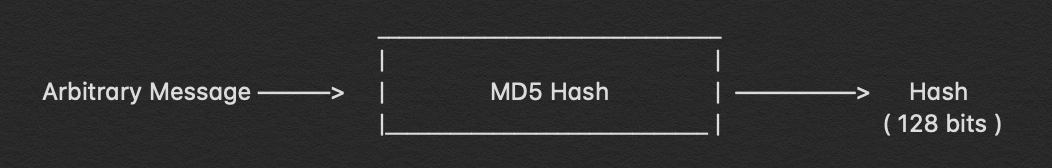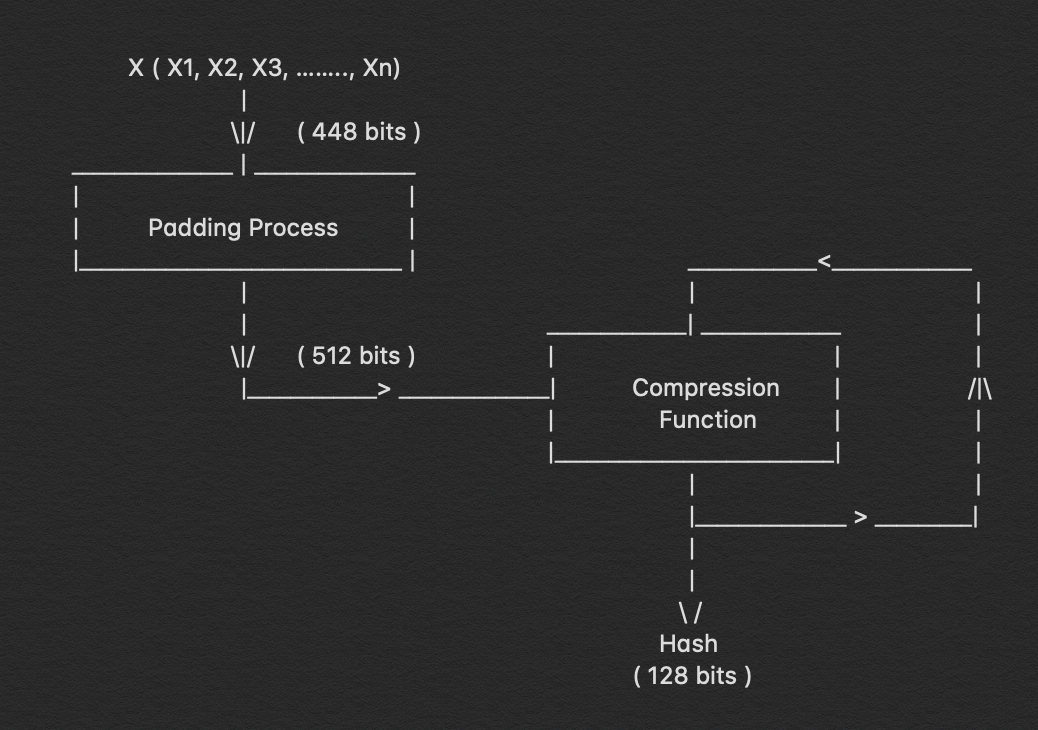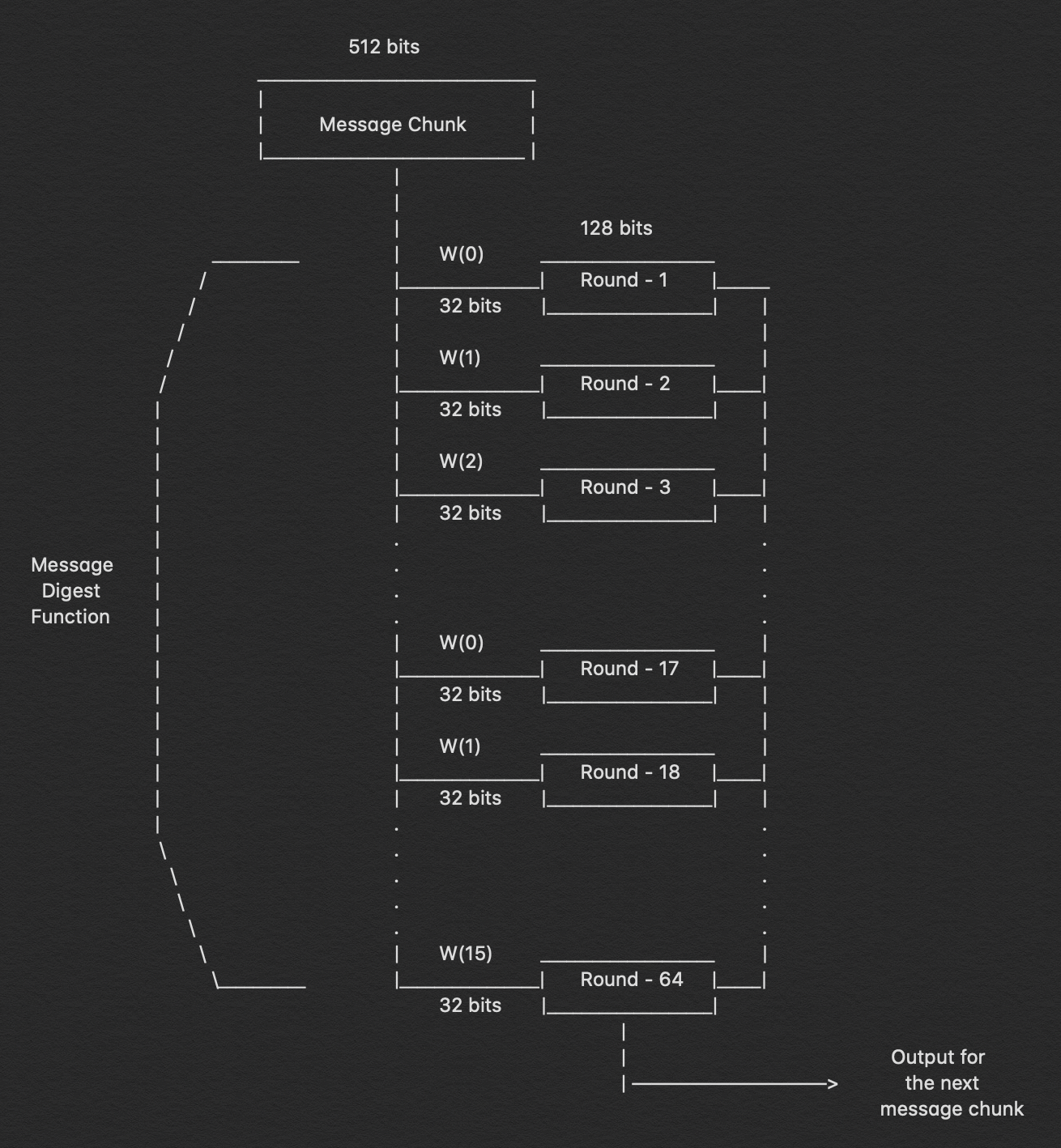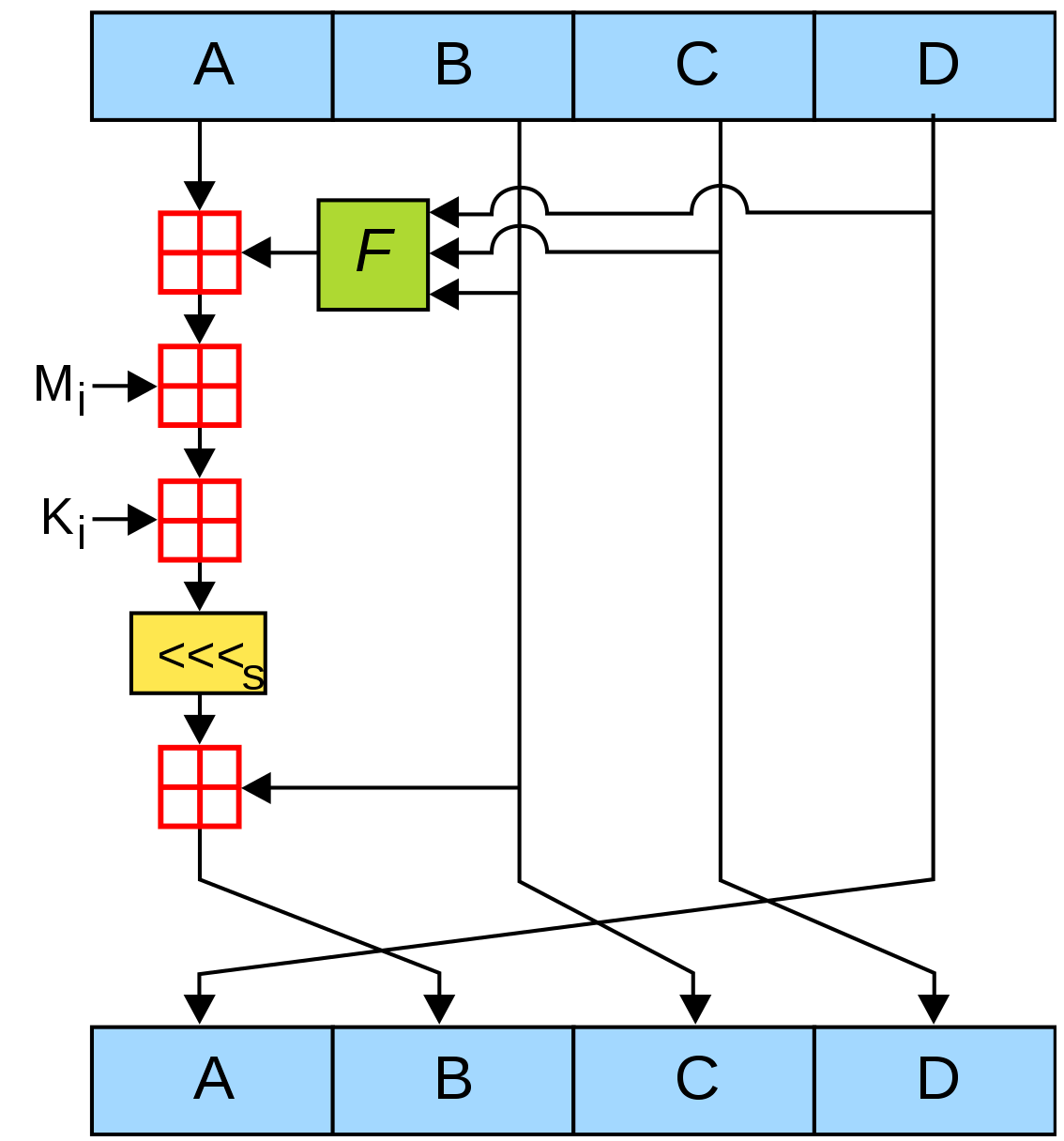Search
•Aditya Anand

# Breaking Down : MD5 Algorithm

#### Looking under the hood and understanding how it works?The previous article that I wrote was on Breaking Down : SHA-1 Algorithm. I have explained the use and purpose of hashing over there, do have a look at it. After writing that I planned why not to write a whole series of the article explaining different hashing algorithms and maybe after that even some cryptographic algorithms and their functioning. One of the major problems in the cybersecurity community is that as we get deep into security and we gradually lose touch with how these algorithms actually work and end up only downloading and using these libraries without giving a second thought about its functionality.This article is an attempt for me to explain the functionality of these hashing algorithms to those who are starting out or those who want to review the basics once again. Hashes are one of the most frequently used terms used in the cybersecurity domain and are extremely useful for various tasks like checksum, file integration verification, password verification etc.

Here is a bird’s eye view of the entire hashing algorithm of how.### Let’s begin!

MD5 hashing technique is where SHA-1 technique has arrived from hence these two are extremely similar. There are some more details I have mentioned over there that will make it easier for you to understand this entire functioning, so you can read that as well - Breaking Down : SHA-1 Algorithm

Ok, now that you have read it let’s go through this whole thing and understand the functioning of MD5 hashing.

Let’s add a few amounts of bits to the message so that it becomes equivalent to 64 bits less than a multiple of 512. The addition of padding bits works in the form that we append 1 to the end of the message and then the rest of the bits that need to be added are 0.

#### 2. Append length bits

This is the step in which we add the remaining 64 bits to the message so that the length of the message becomes an exact multiple of 512. The bits that we add here depends on the length of message ( original one without the padding ) if the length of that message is 8 then we add 1 in the first eight bits and for the next fifty-six bits we add 0, if the length was 64 then all the 64 bits are 1 and if the length is greater than 64 then we calculate the modulus and append that many 1’s and the rest of the 64 bits will be 0’s

#### 3. Initialise MD buffer

This is one of the most important steps of all where we have four different buffers ( A, B, C & D ) and each one of them is 32 bits long.

Their initial default values ( little-endian ):

```A = 0x67452301
B = 0xefcdab89
D = 0x10325476```

#### 4. Process each 512-bit block

Now, let’s go back to our initial discussion where we saw that we perform a total of 64 operations that we perform on each of the 512-bit chunks. These operations that we perform is divided into 4 rounds and 16 operations in each of those rounds. The image below gives us a pictorial representation of the entire compression function i.e. the entire 64 operations.The peculiarity of each of these rounds is that in every round there are unique functions depicted below

```F(B,C,D) = (B AND D) OR ((NOT B) AND D)
G(B,C,D) = (B AND D) OR (C AND (NOT D))
H(B,C,D) = B XOR C XOR D
I(B,C,D) = C XOR (B OR (NOT D))```

For the first round, which consists of 16 operations we will use the F(B,C,D), then we will use G(B,C,D), then H(B,C,D) and for the last round I(B,C,D).

The 512 bit message chunk id is further divided into 16 parts, each of them is of 32 bits, we refer to them as M(1), M(2) and so on. We have a fixed value K(i) which is unique for each operation i.e. there are 64 K(i), mentioned below ( little-endian ).

```K[ 0.. 3] := { 0xd76aa478, 0xe8c7b756, 0x242070db, 0xc1bdceee }
K[ 4.. 7] := { 0xf57c0faf, 0x4787c62a, 0xa8304613, 0xfd469501 }
K[ 8..11] := { 0x698098d8, 0x8b44f7af, 0xffff5bb1, 0x895cd7be }
K[12..15] := { 0x6b901122, 0xfd987193, 0xa679438e, 0x49b40821 }
K[16..19] := { 0xf61e2562, 0xc040b340, 0x265e5a51, 0xe9b6c7aa }
K[20..23] := { 0xd62f105d, 0x02441453, 0xd8a1e681, 0xe7d3fbc8 }
K[24..27] := { 0x21e1cde6, 0xc33707d6, 0xf4d50d87, 0x455a14ed }
K[28..31] := { 0xa9e3e905, 0xfcefa3f8, 0x676f02d9, 0x8d2a4c8a }
K[32..35] := { 0xfffa3942, 0x8771f681, 0x6d9d6122, 0xfde5380c }
K[36..39] := { 0xa4beea44, 0x4bdecfa9, 0xf6bb4b60, 0xbebfbc70 }
K[40..43] := { 0x289b7ec6, 0xeaa127fa, 0xd4ef3085, 0x04881d05 }
K[44..47] := { 0xd9d4d039, 0xe6db99e5, 0x1fa27cf8, 0xc4ac5665 }
K[48..51] := { 0xf4292244, 0x432aff97, 0xab9423a7, 0xfc93a039 }
K[52..55] := { 0x655b59c3, 0x8f0ccc92, 0xffeff47d, 0x85845dd1 }
K[56..59] := { 0x6fa87e4f, 0xfe2ce6e0, 0xa3014314, 0x4e0811a1 }
K[60..63] := { 0xf7537e82, 0xbd3af235, 0x2ad7d2bb, 0xeb86d391 }```

We also carry out left bit rotation in each of the operations and their is an amount set for every operation of every function. The bits we need to rotate left by is depicted by ‘s’. The values of s for each operation are mentioned below.

```s[ 0..15] := { 7,12,17,22,7,12,17,22,7,12,17,22,7,12,17,22 }
s[16..31] := { 5,9,14,20,5,9,14,20,5,9,14,20,5,9,14,20 }
s[32..47] := { 4,11,16,23,4,11,16,23,4,11,16,23, 4,11,16,23 }
s[48..63] := { 6,10,15,21,6,10,15,21,6,10,15,21,6,10,15,21 }```

Now, that we have the values that are required to carry out each operation we can focus on each and every round and how they function.

#### 5. Output message-digest

The image here shows how every operation takes place. Inside every operation, there are again a set of functions that are performed which provides us with the output which in turn acts as inputs for the next operation.We carry out these operations over and over again till we reach the last chunk of the 512 bit message and so the output that we obtain after processing the last chunk is the actual MD5 hash which id of 128 bits, as each A, B, C & D is of 32 bits each and combined together they form the total 128 bits.

### Conclusion

The MD5 hashing algorithm has already been broken down and it basically should not be used at any place like banking and e-commerce websites. Have a look at the entire working of the MD5 hashing let’s go through it once again.

The message that needs to be hashed is first broken down into 448 bits of pieces and for the last piece we carry out padding. An extra 64 bits is appended to it taking the total number of bits to be 512 bits, this acts as a message block going ahead. This message block of 512 bits is broken down in 16 parts of 32 bits each. which then acts as input for the operation that we carry out in the next step. Now let’s get into the main part of the hashing algorithm, there are a total of 64 operations that are performed on the 512 bits message block. These operations are initiated with a default value that I mentioned above ( A, B, C & D). There is a set of functions that are performed in each of these operations and the functions are already defined and the values which it is going to use. The 64 operations that we perform are also divided into 4 different rounds Each round has distinct set of functions, The 32 bit part of the message that we have broken down acts as an input ( depicted in above image ) and there are predefined values of K(i) as well, already mentioned above, the next step is to perform rotate left function, the number of bits the program rotates left is already defined as well. When an entire operation is done it passes on its values to the next operation. After 64 such operations are performed on the first 512-bit message block the output is then passed on for the next operation to be carried out on the next 512 bits till the last message block is reached. The output we receive from the operations being performed on the last message block is the hash of the original message of 128 bits.

So that’s the short version of the whole functioning of the MD5 algorithm.

If you enjoyed it please do clap & let’s collaborate. Get, Set, Hack!